# How to compare two columns in Excel for matches and differences

Comparing columns in Excel is something that we all do once in a while. Microsoft Excel offers a number of options to compare and match data, but most of them focus on searching in one column. In this tutorial, we will explore several techniques to compare two columns in Excel and find matches and differences between them.

## How to compare 2 columns in Excel row-by-row

When you do data analysis in Excel, one of the most frequent tasks is comparing data in each individual row. This task can be done by using the IF function, as demonstrated in the following examples.

### Example 1. Compare two columns for matches or differences in the same row

To compare two columns in Excel row-by-row, write a usual IF formula that compares the first two cells. Enter the formula in some other column in the same row, and then copy it down to other cells by dragging the fill handle (a small square in the bottom-right corner of the selected cell). As you do this, the cursor changes to the plus sign: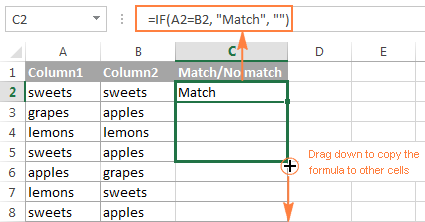#### Formula for matches

To find cells within the same row having the same content, A2 and B2 in this example, the formula is as follows:

`=IF(A2=B2,"Match","")`

#### Formula for differences

To find cells in the same row with different values, simply replace the equals sign with the non-equality sign (<>):

`=IF(A2<>B2,"No match","")`

#### Matches and differences

And of course, nothing prevents you from finding both matches and differences with a single formula:

`=IF(A2=B2,"Match","No match")`

Or

`=IF(A2<>B2,"No match","Match")`

The result may look similar to this: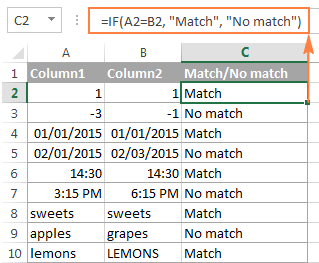As you see, the formula handles numbers, dates, times and text strings equally well.

Tip. You can also compare two columns row-by-row using Excel Advanced Filter. Here is an example showing how to filter matches and differences between 2 columns.

### Example 2. Compare two lists for case-sensitive matches in the same row

As you have probably noticed, the formulas from the previous example ignore case when comparing text values, as in row 10 in the screenshot above. If you want to find case-sensitive matches between 2 columns in each row, then use the EXACT function:

`=IF(EXACT(A2, B2), "Match", "")`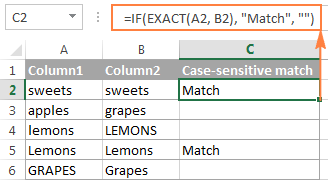To find case-sensitive differences in the same row, enter the corresponding text ("Unique" in this example) in the 3rd argument of the IF function, e.g.:

`=IF(EXACT(A2, B2), "Match", "Unique")`

## Compare multiple columns for matches in the same row

In your Excel worksheets, multiple columns can be compared based on the following criteria:

• Find rows with the same values in all columns (Example 1)
• Find rows with the same values in any 2 columns (Example 2)

### Example 1. Find matches in all cells within the same row

If your table has three or more columns and you want to find rows that have the same values in all cells, an IF formula with an AND statement will work a treat:

`=IF(AND(A2=B2, A2=C2), "Full match", "")`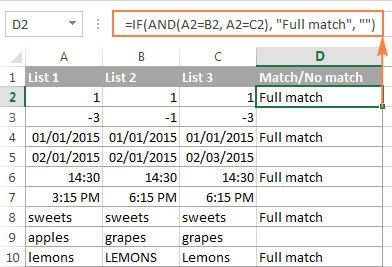If your table has a lot of columns, a more elegant solution would be using the COUNTIF function:

`=IF(COUNTIF(\$A2:\$E2, \$A2)=5, "Full match", "")`

Where 5 is the number of columns you are comparing.

### Example 2. Find matches in any two cells in the same row

If you are looking for a way to compare columns for any two or more cells with the same values within the same row, use an IF formula with an OR statement:

`=IF(OR(A2=B2, B2=C2, A2=C2), "Match", "")`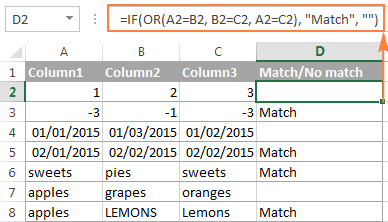In case there are many columns to compare, your OR statement may grow too big in size. In this case, a better solution would be adding up several COUNTIF functions. The first COUNTIF counts how many columns have the same value as in the 1st column, the second COUNTIF counts how many of the remaining columns are equal to the 2nd column, and so on. If the count is 0, the formula returns "Unique", "Match" otherwise. For example:

`=IF(COUNTIF(B2:D2,A2)+COUNTIF(C2:D2,B2)+(C2=D2)=0,"Unique","Match")`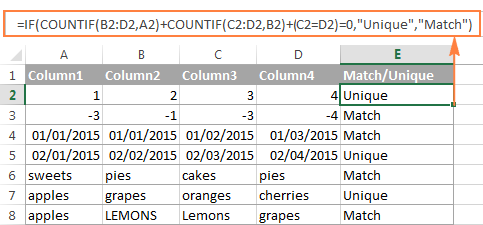## How to compare two columns in Excel for matches and differences

Suppose you have 2 lists of data in Excel, and you want to find all values (numbers, dates or text strings) which are in column A but not in column B.

For this, you can embed the COUNTIF(\$B:\$B, \$A2)=0 function in IF's logical test and check if it returns zero (no match is found) or any other number (at least 1 match is found).

For instance, the following IF/COUNTIF formula searches across the entire column B for the value in cell A2. If no match is found, the formula returns "No match in B", an empty string otherwise:

`=IF(COUNTIF(\$B:\$B, \$A2)=0, "No match in B", "")`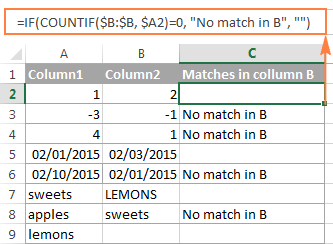Tip. If your table has a fixed number of rows, you can specify a certain range (e.g. \$B2:\$B10) rather than the entire column (\$B:\$B) for the formula to work faster on large data sets.

The same result can be achieved by using an IF formula with the embedded ISERROR and MATCH functions:

`=IF(ISERROR(MATCH(\$A2,\$B\$2:\$B\$10,0)),"No match in B","")`

Or, by using the following array formula (remember to press Ctrl + Shift + Enter to enter it correctly):

`=IF(SUM(--(\$B\$2:\$B\$10=\$A2))=0, " No match in B", "")`

If you want a single formula to identify both matches (duplicates) and differences (unique values), put some text for matches in the empty double quotes ("") in any of the above formulas. For example:

`=IF(COUNTIF(\$B:\$B, \$A2)=0, "No match in B", "Match in B")`

## How to compare two lists in Excel and pull matches

Sometimes you may need not only match two columns in two different tables, but also pull matching entries from the lookup table. Microsoft Excel provides a special function for this - the VLOOKUP function. As an alternative, you can use a more powerful and versatile INDEX MATCH formula. The users of Excel 2021 and Excel 365, can accomplish the task with the XLOOKUP function.

For example, the following formulas compare the product names in columns D against the names in column A and pull a corresponding sales figure from column B if a match is found, otherwise the #N/A error is returned.

`=VLOOKUP(D2, \$A\$2:\$B\$6, 2, FALSE)`

`=INDEX(\$B\$2:\$B\$6, MATCH(\$D2, \$A\$2:\$A\$6, 0))`

`=XLOOKUP(D2, \$A\$2:\$A\$6, \$B\$2:\$B\$6)`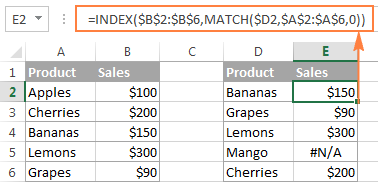If you don't feel very comfortable with formulas, you can have the job done using a fast and intuitive solution - Merge Tables Wizard.

## Compare two lists and highlight matches and differences

When you compare columns in Excel, you may want to "visualize" the items that are present in one column but missing in the other. You can shade such cells in any color of your choosing by using the Excel Conditional Formatting feature and the following examples demonstrate the detailed steps.

### Example 1. Highlight matches and differences in each row

To compare two columns and Excel and highlight cells in column A that have identical entries in column B in the same row, do the following:

• Select the cells you want to highlight (you can select cells within one column or in several columns if you want to color entire rows).
• Click Conditional formatting > New Rule… > Use a formula to determine which cells to format.
• Create a rule with a simple formula like `=\$B2=\$A2` (assuming that row 2 is the first row with data, not including the column header). Please double check that you use a relative row reference (without the \$ sign) like in the formula above.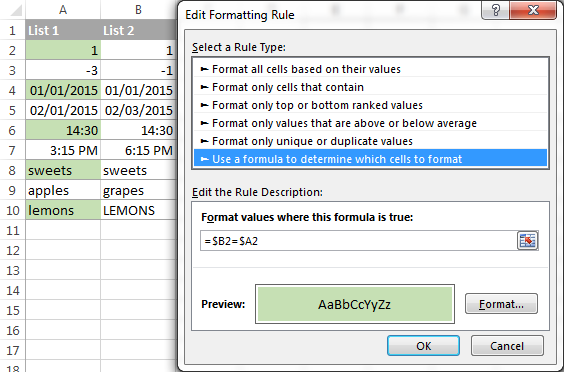To highlight differences between column A and B, create a rule with this formula:

`=\$B2<>\$A2`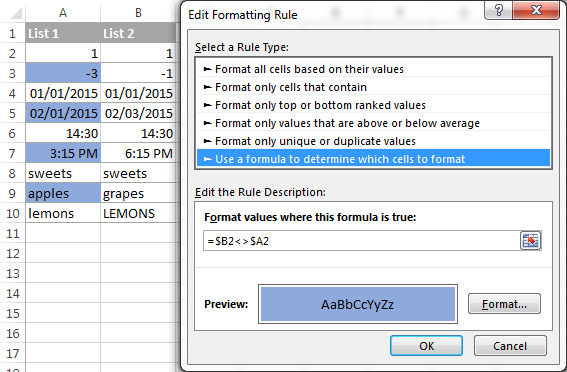If you are new to Excel conditional formatting, please see How to create a formula-based conditional formatting rule for step-by-step instructions.

### Example 2. Highlight unique entries in each list

Whenever you are comparing two lists in Excel, there are 3 item types that you can highlight:

• Items that are only in the 1st list (unique)
• Items that are only in the 2nd list (unique)
• Items that are in both lists (duplicates) - demonstrated in the next example.

This example demonstrates how to color the items that are only in one list.

Supposing your List 1 is in column A (A2:A6) and List 2 in column C (C2:C5). You create the conditional formatting rules with the following formulas:

Highlight unique values in List 1 (column A):

`=COUNTIF(\$C\$2:\$C\$5, \$A2)=0`

Highlight unique values in List 2 (column C):

`=COUNTIF(\$A\$2:\$A\$6, \$C2)=0`

And get the following result: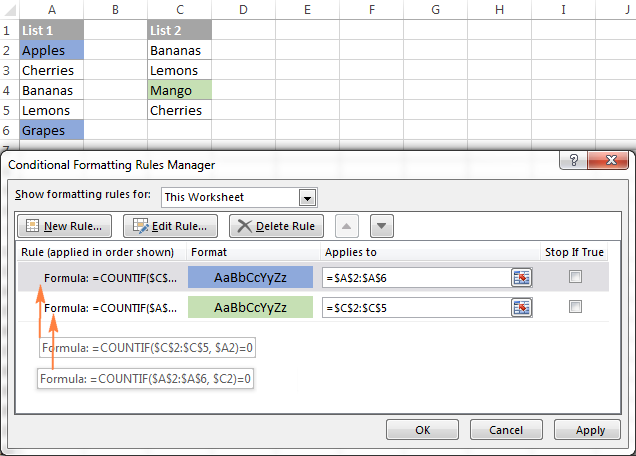### Example 3. Highlight matches (duplicates) between 2 columns

If you closely followed the previous example, you won't have difficulties adjusting the COUNTIF formulas so that they find the matches rather than differences. All you have to do is to set the count greater than zero: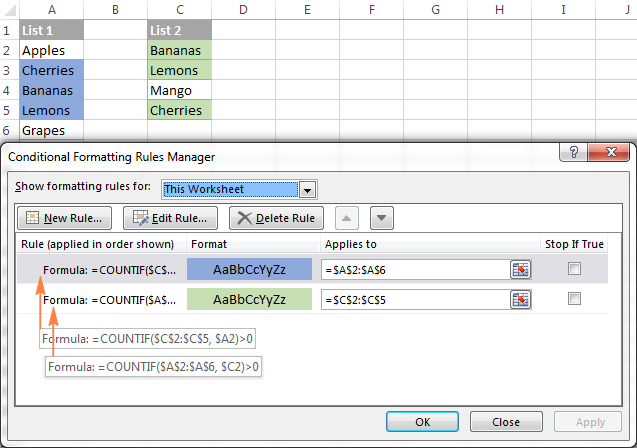Highlight matches in List 1 (column A):

`=COUNTIF(\$C\$2:\$C\$5, \$A2)>0`

Highlight matches in List 2 (column C):

`=COUNTIF(\$A\$2:\$A\$6, \$C2)>0`

## Highlight row differences and matches in multiple columns

When comparing values in several columns row-by-row, the quickest way to highlight matches is creating a conditional formatting rule, and the fastest way to shade differences is embracing the Go To Special feature, as demonstrated in the following examples.

### Example 1. Compare multiple columns and highlight row matches

To highlight rows that have identical values in all columns, create a conditional formatting rule based on one of the following formulas:

`=AND(\$A2=\$B2, \$A2=\$C2)`

or

`=COUNTIF(\$A2:\$C2, \$A2)=3`

Where A2, B2 and C2 are the top-most cells and 3 is the number of columns to compare.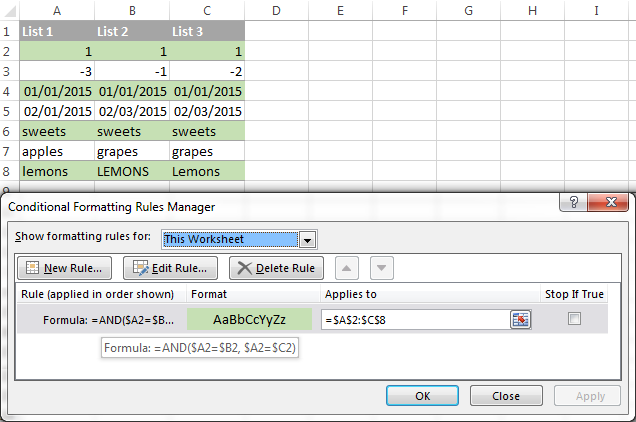Of course, neither AND nor COUNTIF formula is limited to comparing only 3 columns, you can use similar formulas to highlight rows with the same values in 4, 5, 6 or more columns.

### Example 2. Compare multiple columns and highlight row differences

To quickly highlight cells with different values in each individual row, you can use Excel's Go To Special feature.

1. Select the range of cells you want to compare. In this example, I've selected cells A2 to C8.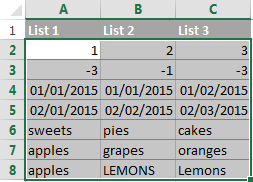By default, the top-most cell of the selected range is the active cell, and the cells from the other selected columns in the same row will be compared to that cell. As you can see in the screenshot above, the active cell is white while all other cells of the selected range are highlighted. In this example, the active cell is A2, so the comparison column is column A.

To change the comparison column, use either the Tab key to navigate through selected cells from left to right, or the Enter key to move from top to bottom.

Tip. To select non-adjacent columns, select the first column, press and hold Ctrl, and then select the other columns. The active cell will be in the last column (or in the last block of adjacent columns). To change the comparison column, use the Tab or Enter key as described above.

2. On the Home tab, go to Editing group, and click Find & Select > Go To Special… Then select Row differences and click the OK button.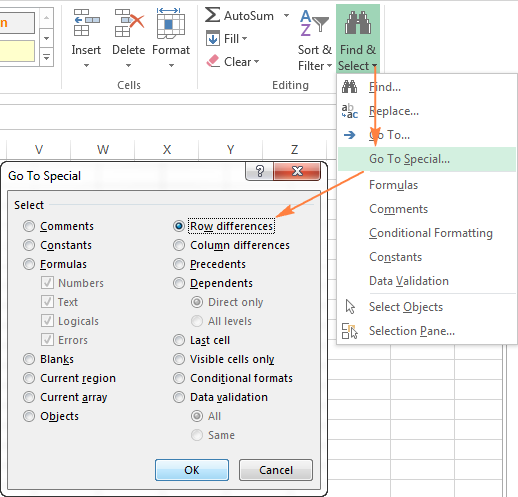3. The cells whose values are different from the comparison cell in each row are colored. If you want to shade the highlighted cells in some color, simply click the Fill Color icon on the ribbon and select the color of your choosing.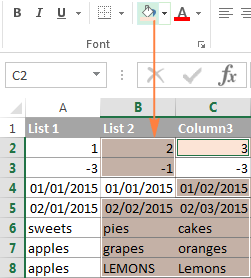## How to compare two cells in Excel

In fact, comparing 2 cells is a particular case of comparing two columns in Excel row-by-row except that you don't have to copy the formulas down to other cells in the column.

For example, to compare cells A1 and C1, you can use the following formulas.

For matches:

`=IF(A1=C1, "Match", "")`

For differences:

`=IF(A1<>C1, "Difference", "")`

To learn a few other ways to compare cells in Excel, please see:

## Formula-free way to compare two columns / lists in Excel

Now that you know Excel's offerings for comparing and matching columns, let me show you our own solution for this task. This tool is named Compare Two Tables and it is included in our Ultimate Suite.

The add-in can compare two tables or lists by any number of columns and both identify matches/differences (as we did with formulas) and highlight them (as we did with conditional formatting).

For the purpose of this article, we'll be comparing the following 2 lists to find common values that are present in both.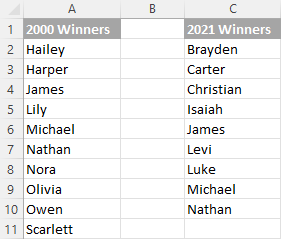To compare two lists, here are the steps you need to follow:

1. Start with clicking the Compare Tables button on the Ablebits Data tab.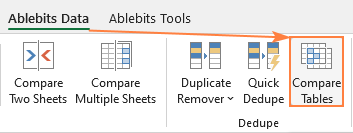2. Select the first column/list and click Next. In terms of the add-in, this is your Table 1.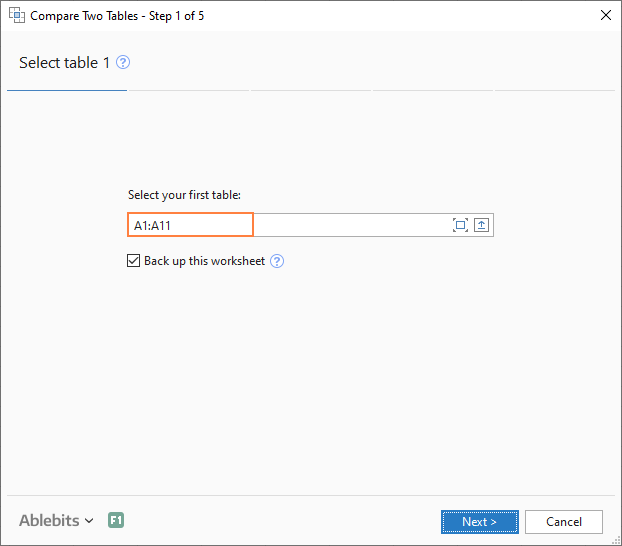3. Select the second column/list and click Next. In terms of the add-in, it is your Table 2, and it can reside in the same or different worksheet or even in another workbook.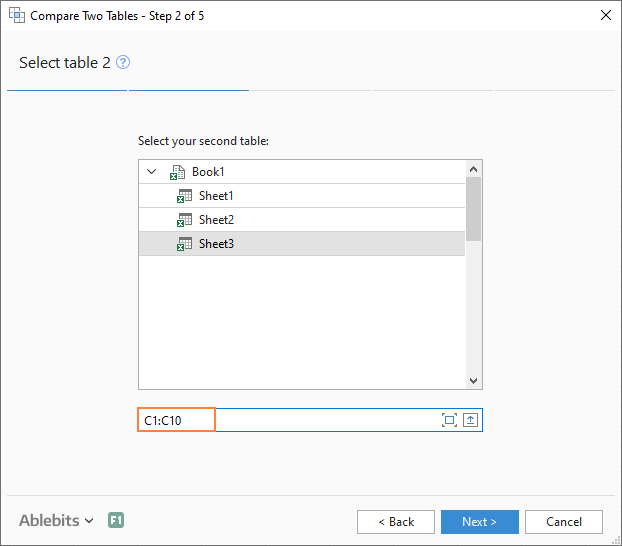4. Choose what kind of data to look for:
• Duplicate values (matches) - the items that exist in both lists.
• Unique values (differences) - the items that are present in list 1, but not in list 2.

Since our aim is to find matches, we select the first option and click Next.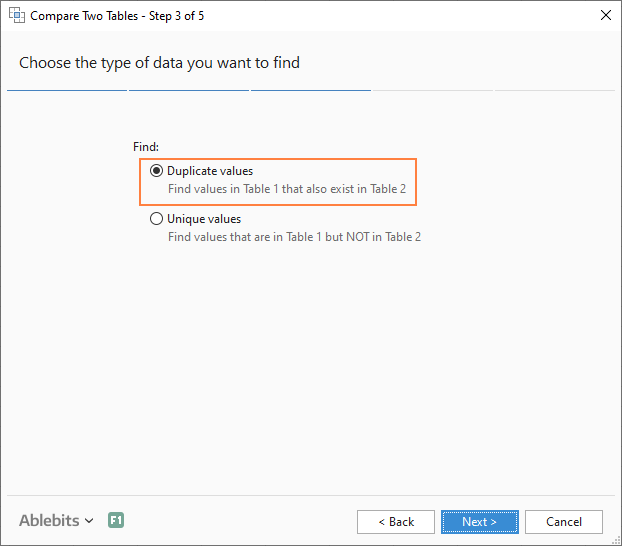5. This is the key step where you select the columns for comparison. In our case, the choice is obvious as we are only comparing 2 columns: 2000 Winners against 2021 Winners. In bigger tables, you can select several column pairs to compare by.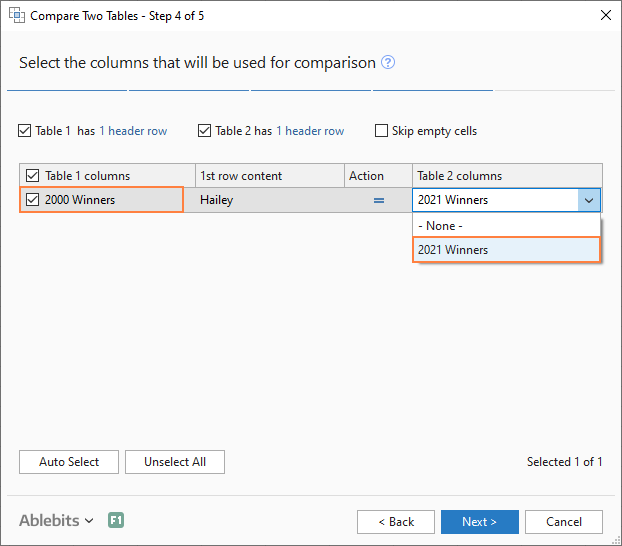6. In the final step, you choose how to deal with the found items and click Finish.

A few different options are available here. For our purposes, these two are most useful:

• Highlight with color - shades matches or differences in the selected color (like Excel conditional formatting does).
• Identify in the Status column - inserts the Status column with the "Duplicate" or "Unique" labels (like IF formulas do).

For this example, I've decided to highlight duplicates in the following color: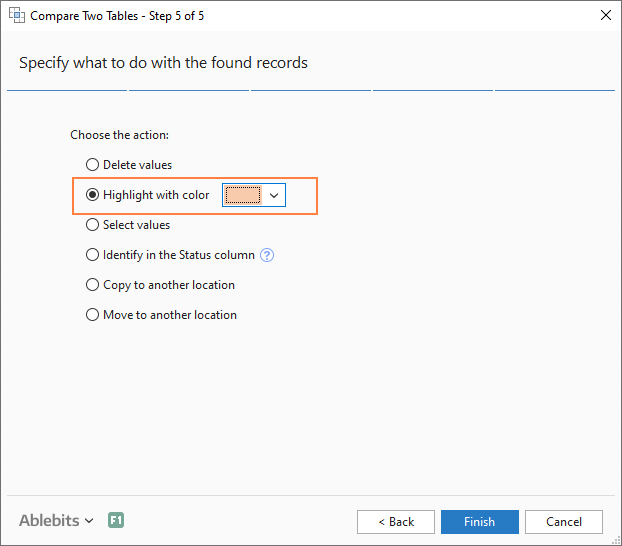And in a moment, got the following result: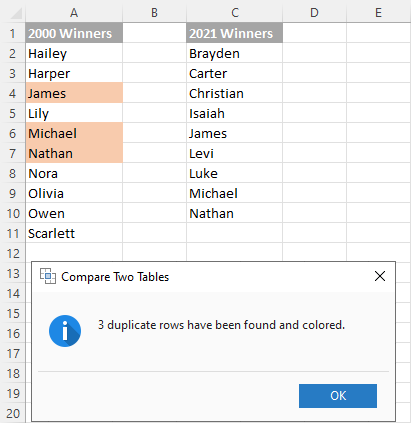With the Status column, the result would look as follows: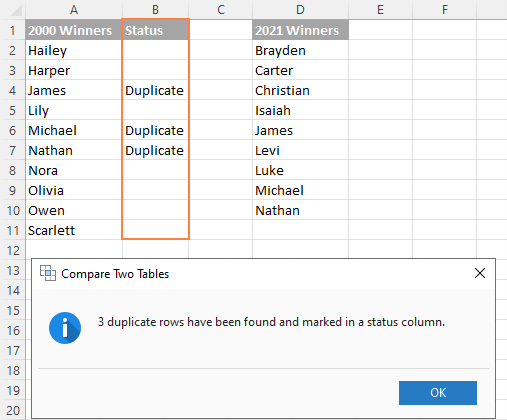Tip. If the lists you are comparing are in different worksheets or workbooks, it might be helpful to view Excel sheets side by side.

This is how you compare columns in Excel for matches (duplicates) and differences (unique values). If you are interested to try this tool, you are welcome to download an evaluation version using the below link.

I thank you for reading and encourage you to check out other helpful tutorials that we have :)

Compare Excel Lists - examples (.xlsx file)
Ultimate Suite - trial version (.exe file)

## You may also be interested in

1. Need a formula based on below criteria:
I have two cells, A and B,
if the difference btw A & B is less than 6 or equal to 6, "No Change"
if greater than 6 and less than 9, "Slight Change"
if greater than 10 "Significant Change"

Also the formula should override the difference with positive numbers, even the B is larger than A, vice-versa

2. I would like to know how to compare a range "A1" of file names with a directory and return the date the file was last save to a each matching cell in range "B1"

3. Excellent website, thanks.

4. Is it possible to compare for certain values two different sheets of same Ms Excel file?

5. Hello good evening,

I need small help. So I need to get how many same numbers repeats in Column A that matches same value in column B. Example How many times 38692 repeats in column A and has value of \$5 in column B? Can anyone help me with that please
A B
38770 \$10.00
38692 \$5.00
38692 \$5.00
38692 \$5.00
38692 \$10.00
38769 \$10.00
38692 \$5.00
38692 \$5.00
38692 \$10.00
\$0.00
\$0.00
38694 \$10.00
\$0.00
38694 \$10.00
\$0.00

6. I need a formula to count how many times a specific range of numbers occurs twice in a row.

Example: how many times a number between 1 and 9 occurs at the same time in column C and D

1, 7, 9, 14, 20, 27
1, 3, 4, 5, 14, 21,
2, 3, 4, 17, 22, 23
4, 5, 7, 8, 20, 24
16, 17, 18, 20, 21, 22

I thought I could use:
=COUNTIF(C1:C379,"<=9") + COUNTIF(D1:D379,<=9")

But it is adding the number of times the range occurs in column c to the number of times the range occurs in column D. What I need is the number of times the range occurs in column C and D within the same row, or in other words, at the same time.

Thanks!

7. Very helpful. Thanks so much!

8. how to convert excel data to note pad text transpose (T). and need to add some ad some special character with the text

9. Hello,
I am trying to do a comparison between 2 columns for values only. In one column, the value is 13 while the other I am comparing it to is 13.0. None of the methods I have tried return a match.
Is there a way to compare for a match in value of the two without having to reformatting the columns to match?
Thank you in advance for any help that can be provided.

10. Thanx. it helped me.

11. I have some items and rates in one table, other table I need to type item name and get relevant rate to next column in same row Table1 Table2 Item. Rate. Item. Rate. A. 3. D. ? B. 4. A. ? C. 6. B. ? D. 4. E. 1. .... Z. 4.

12. Hi ,

How to compare two cells whether another cell contains same word,

Location Code Description Location from part no Status
LEAF SPRING FRONT REAR AXLE PARABOLIC SPRING FALSE

Thanks

• Hello E Sivakumar,

Please, you can use the following formula:
=IF(A1=B1,"match", "no match")

13. Hello,
I have two data sets (sheets), first columns in both the sheets have data that can be matched. Based on the first columns, I want to create a new data set having the expanded data for each of the columns.

Thanks.

14. Hi- Thanks for your post. I have another requirement.
I have two columns ex A and B. In col A is a list of employees and Col B is list of employees (kind of sub set of Col A), Col B can contain all the employees present in Col A, but if any employee left in this month then that employees name will be removed from Col B. Also Col B can contain new employees as well.

So end of the month I want to run a report and show that Which all employees Of col A is present in Col B , list them in a new col C, and also Column C also should list out if any new employees names are found in column B and put that also in Column C.

Can you help?

15. I have 2 sheets. 1 has a column with 14 digit numbers representing products. The 2nd sheet has a column with 10 digit numbers also representing products. Product descriptions are different in each sheet. I want to verify the list of 10 digit numbers are included within the sheet with 14 digit numbers. Can you help? Thanks!

• Hello Jen,

For us to be able to assist you better, please send us a small sample table with your data in Excel and include the expected result. Thank you.

16. Thanks a lot,
It saved me couple of hours.

17. I have a Google spreadsheet with 50 separate titled sheets with one that I have labeled as Forms_Received. All sheets are different meetings that we have going on and I need to compare a column in all the sheets with the Forms_Received sheet and have a column in all the sheets marked with "Y" if a form was received on each of the sheets. I would prefer that the "Y" be an exact match from the column in the Forms_Received sheet, since it will have a linked document attached to the "Y". What formula should I use? If you need to see what I am talking about, please let me know.
Thank you,

18. I need a formula to count how many times column A has a ON and NS that represents a CU in column B. In the case below ON and NS represent a total of 3 CU's in column B

A B
ON CU
BC CU
AB CU
ON CU
NS CU
NS BA

Eg. =+COUNTIFS(QtradePartners!B3:B136,"=ON",QtradePartners!B3:B136,"=NS",QtradePartners!C3:C136,"=CU") ... THIS IS NOT WORKING and I think it makes sense that it doens't work... Anyone help?

• Hello Marc,

Please, you can use the following formula:
=COUNTIFS(A:A,"ON",B:B,"CU") + COUNTIFS(A:A,"NS",B:B,"CU")

19. List 1 List 2
1 a
2 a
3 b
3 b
1 a
3 c
1 d

Hello, please help me in the above example. I want to check if list 1 and List 2 has same row values. Like first row is same as the fifth so row 3 and 4.

WHat formula should I use.

• Hello Ashok,

Please, you can use the following formula:
=IF(COUNTIFS(A:A,A2,B:B,B2)< 2,"no match","match")

20. Hello
I have two excel sheets
One sheets boys sheet mention name, gotra, year, qualification salary
second sheets girls mention name, gotra, year, qualification salary
how we can match it

21. Hi, I have two columns.

column A: a list of 60 different distinct computer hardware in every cell.

Column B: over a thousand combination of not only including from 0 up to 10 computer hardware, but also have brand names in each cell.

All I want to achieve is to display in column C what are those computer hardware A shown up in B, in the same row as in column B.

22. Hi, whuch formula should I use if I want to compare 2 excel sheets. 1 has been revised and 1 has no changed.

• I fully comprehend, by virtue of your tutorial, as to how to compare two excel files for differences. But then I need learn how to compare the two excel files (and also the two/more columns/rows within an excel file) for matches or similar entries.
Thanking you in anticipation.

• i love you.

23. Dear,

How to take data from two columns to another table ie. if we have two columns and having some data true and false so i need to separate true data and false data in another table

24. Hi Guys,

How to check duplication of two numbers in two different column, with the two number provided in different sequence order. sample as in below.

Column A Column B Column C (result)
1234 2341 A=B

25. Yes its working. Thank you so much. Grateful for the support.

26. Thanx for such an informative article over excel,still struggling to find the solution ,Pl help if possible.
I've two columns in which one column say B having exhaustive values,while in another say column A ,some values are missing.How to ascertain either through Vlookup,Countif or If,which values are missing. Here,it is to be noted that all data in A is in column B,but vice versa is not true. Just wanted to know missing data. Pl help in getting formula for the same.Total entries in column A is less than column B(vertically) Plzz..

• Hello Avinash,

If my understanding of the task is correct, you need to identify the values that are present in column B, but not in column A. If so, you can use the following formula:
=IF(COUNTIF(\$A:\$A, \$B2)=0, "missing", "")

Where B2 is the topmost cell with data, ignoring column headers.

When you copy the formula down the column, the word "missing" will appear in the rows containing data in column B that do not appear anywhere in column A. To get a list of missing values, apply Excel's auto-filter, filter the formula column by "missing" and copy the filtered values in column B.

27. Afternoon,
I want to put a conditional format in column B.
I have a target number in column A and input Actual in B.
I want a conditional format in B
if the number is same as or above target in A then "fill" the cell otherwise no fill.

610 0 - no fill
1525 915 - no fill
610 0 - no fill
610 710 - fill
610 610 - fill
305 0 - no fill

28. Sorry,
I posted before completing the question

I want to compare likewise

A B C
Danta,Alex,John,Ryan Alex,Ryan,Danta,John True
John,Alex,Ryan Alex,John,Danta False
Michelle,Rob,Sean Michelle,Rob,Sean True

29. Hello,

I want to know can we compare this type cells

Cell A

30. Hi,
Let say that column A has 2 cells and are as follow:
A1 : 10
A2 : 20
And in column C there are 2 cells as follow:
C1 : 10,20
C2 : 20,10
In cell B1 I want that it searches in A1 cell that is 10 and if the first number in column C is 10(that is C1 and not C2), it copies cell C1 in B1.

31. Hi i currently using index and match function in excel, to auto match and index the value back, if i put a set of new data to compare with my original data.

But however sometime a new data had a multiple result , how to i auto insert a row on the original data columns for the multiple result?

this is my formula code : =INDEX(\$L:\$L,MATCH(\$A:\$A,\$K:\$K,0)) it could match and bring back the index value back i wanted ... but those with multiple result it only return back to the first result it show.

regards istillanoob

32. Hello.. I now actually trying using Index match/IF function to try to index the value from “ColE” onto “ColB” , by matching “ColA” compare with “ColD”.

For my eg.
Shown below “Urr” got 3 different value in “ColE” how to I match and insert the three “Urr” with the different number
result in “ColE” onto “ColA” with the same exact result number should appear in “ColB”???
And “colA” “Usw” data still match with “ColD” “Usw”even is not in the same row?

something like new data replace with original data orderly, plus without deleting original data if the data don not appear in new data.

Eg.

ColA
Doman
Urr
Usw

ColD ColE
Doman 1234
Urr 12345
Urr 12345
Urr 12346

33. if column A has names and column D has dates. Is there a way to highlight equal dates that belong to the same name?

• Hello Jonathon,

You can create a conditional formatting rule with the following formula:

=COUNTIFS(A:A, A1, D:D, D1)>1

34. Somebody to help me....
Dear all,
I have a huge problem...
I have two rows A and B,
Values in column A (3,4,4,5,5,6) in column B (3,3,4,5,6,6), I wont to compare this two column
3 with 3 nothing
4 with 4 nothing
4 with x 4
5 with 5 nothing
5 with x 5
nad reverse (if you dont have in column A, have in column B) also to be in column C.
If you understan (match just once number by number), if aleready match to give me value.
Tnks a lot, to all.

35. Data validation list A =a1:a10
Data validation list b =b1:b10

my problem is if selected list from List A or List B cell D1 change automatically any formula or any solution

36. Hey sir if I'm having 5 cells in 2 columns say column A and Column B.
with entries like.
Column A Column B
1. B21 Rojer
2. B11 Rojer
5. C22 Chris
6. C22 Chris
How can I select the row 1 and row 2 which are having different code for same name Rojer

37. USE THE FN EXACT.. FOR MATCHING TWO CLM

38. Hi

i need to compare one column to all the rows present in Second column.can someone answer?

39. I have a product if statement but it’s not working properly

see below

=IF(SUMPRODUCT((\$A\$2:\$A2=A2)*(\$B\$2:\$B2=B2)*(\$C\$2:\$C2=C2))>1,0,1)

….It’s not providing 0’s where there is repeat data across the columns based on the rows above. If it were working it would place a 0 rather than 1.

Thank you Everyone!

40. That output looked funky so I wanted to clarify:

Col. A = #
Col. B = Name
Col. C = TYPE

Col.D = Nails
with 1 and 0 in data which shows a 1 as a unique listing throughout the rows above and columns A – C.

Col. E = Comedy with the same statement as above with 1’s and 0’s
Col. F = Mane ….
Col. G = Paw ….

41. # Name TYPE
abcd Abbott Nails
abcde Cost Comedy
baco Lion Mane
zhty Bear Paw
zhty Bear Paw

The above is a simplified version of my data however there are numerous rows and columns but overall I would like to identify unique values across columns and rows simultaneously by documenting it with a 1 as unique or a 0 as it occurred in previous rows across columns.

# Name TYPE Nails Comedy Mane Paw
abcd Abbott Nails 1 0 0 0
abcde Cost Comedy 0 1 0 0
abcde Cost Comedy 0 0 0 0
baco Lion Mane 0 1 0 0
baco Lion Mane 0 0 0 0
zhty Bear Paw 0 0 0 1
zhty Lion Mane 0 0 1 1

42. Thanks for tutorial. The comparing can be done with Excel Vba Codes .The compare process can be made with Excel Vba Worksheet.Countif Function .

Two columns in different worksheets were compared in this template .Found different results as entire row were copied to second worksheet .
Codes :
"Dim stk, msb As Worksheet
Set stk = Sheets("Page1")
Set msb = Sheets("Page2")

Application.ScreenUpdating = False
sat = (msb.Range("A" & Rows.Count).End(xlUp).Row) + 1
For i = 2 To stk.Range("A" & Rows.Count).End(xlUp).Row
If WorksheetFunction.CountIf(msb.Range("A2:A" & msb.Range("A" & Rows.Count).End(xlUp).Row), stk.Cells(i, "A")) = 0 Then
msb.Range("a" & sat).EntireRow.Value = stk.Range("a" & i).EntireRow.Value
msb.Range("a" & sat).Interior.ColorIndex = 22
sat = sat + 1
End If
Next
...
"
Template at :http://goo.gl/ZeOwa2

43. Hi,

your description is actually very clear and helpful,thanks a lot. But with my data set it didn´t work for some reason. I want to compare two columns with gene names to find genes which are in column A and in column B, so i tried your example "Example 3. Highlight matches (duplicates) between 2 columns" respectively "Example 2. Highlight unique entries in each list", but unfortunately no cells were marked although there are duplicates. Do you have any idea why this happened?

Thanks a lot!!

44. I've been using a column compare macro which gives me the data (which is there in column A but not in column b) into column C AND the data (which is there column B but not in column A) into column D. I'm trying to find out the formula used to make this macro.

Thanks!

45. kindly help me to assign the remarks of attendence as excellent, good or bad based on the student attendence. It will be mentioned in range as 95-99 as Excellent,90-94 as good, 85-89 as average and like.

• Hi Manoj,

You need nested IF functions for this. For example:
=IF(A2>=95, "Excellent", IF(A2>=90, "Good", IF(A2>=85, "Average", "Poor ")))

46. I have a spreadsheet that needs to be alpha dominant where column A is a lower alpha character and column B wants to be a higher alpha character relative to column A.
An example of this is.
Column A Column B
ABC DEF

However, if the columns A and B values are flipped where it is not the correct output such as will be incorrect.
Column A Column B
DEF ABC

I need a formula to weed out the lower alpha to higher alpha issue on a spreadsheet with thousands of rows.

Thanks

I need to compare every to cells in a column that belongs to same object in 2 rows.
I mean for each object (out of many objects) I have 2 rows and multiple columns and for every each of this columns I need to compare every 2 cells (that are one row after another) to see if the data is the same, if not then I need to highlight the 2 cells.
I hope someone can understand me and answer me. I need some condition or maybe to write a command in Macro.

48. I have large amount of data,

Example
COL-A " PIPE SEAMLESS API 5L Gr. B PSL2 NACE+HIC 3/4"(20 MM) SCH 80 PLAIN END ASME B36.10M "

COL B- " PIPE SEAMLESS API 5L GR B PSL2 NACE + HIC 0.75 SCH 80 PLAIN END ASME B36.10M "

I want to find the difference in content of cell,
e.g in example difference between COL-A & COL-B is {3/4"(20 MM) & 0.75}

Can anyone help?

49. Hi,

I have 1 spreadsheet with 4 separete text tables. Each table contains a code on the first column and a description on the second.

How can I highlight the mtaching letters sequence or identical words on the second column (description) of two considered tables?

50. I have 2 excel worksheets. 1st sheet contain 900 names with details in 9 columns.
2nd sheet is with 600 names (which are available in above 900) but with different details in 6 columns.
I want to merge the details of 2nd list of 600 with those details of 600 available in the 900 name list.
How to do this.